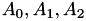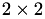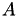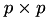HLIBpro  2.4
Block Matrix Assembly

When constructing 𝓗-matrices consisting of several differently defined subblocks, e.g., the block diagonalout of given matrices, 𝓗𝖫𝖨𝖡𝗉𝗋𝗈 supports two different methods.

# Using assemble_block

The function

std::unique_ptr< TMatrix >
assemble_block ( const size_t block_rows,
const size_t block_cols,
TMatrix ** submatrices,
const bool copy );

implements a direct way for assembling a new block matrix out of a given set of (sub-) matrices.

Here, block_rows and block_cols define the number of block rows and block columns of the new matrix while submatrices stores pointers to the corresponding subblocks in column wise ordering. If the parameter copy is true, a copy of each subblock is stored in the new matrix instead.

A basic example on the usage of assemble_block for constructing amatrix is

TMatrix * A_00 = ...;
TMatrix * A_01 = ...;
TMatrix * A_10 = ...;
TMatrix * A_11 = ...;
TMatrix * submatrices[] = { A_00, A_10, A_01, A_11 }; // column-wise !
auto A = assemble_block( 2, 2, submatrices, false );

For the function to work properly, the cluster trees of all subblocks have to be compatible, e.g., all subblocks in the same block column must be defined using the same column cluster tree. The same holds for the row cluster tree of all subblocks in a block row.

Upoin construction of, the index sets of all subblocks are adjusted to be numbered consecutively, e.g., A_00->row_is().last()+1 == A_01->row_is().first().

Subblocks may also be NULL if the corresponding block does not exists, e.g., as in the above block diagonal example.

If all subblocks are of type THMatrix, the new matrix is also constructed as an 𝓗-matrix. In particular, the new matrix will store matching permutations based upon the permutations of the given subblocks, which is important for importing/exporting data, e.g., RHS vectors. Furthermore, in this case all subblocks will loose the permutation information and will be reduced to standard TBlockMatrix objects.

## C interface function

The function assemble_block is also available in the C interface:

hlib_matrix_t
hlib_matrix_assemble_block ( const size_t block_rows,
const size_t block_cols,
hlib_matrix_t * submatrices,
const int copy,
int * info );

The above example for ablock matrix would look as

hlib_matrix_t A_00 = ...;
hlib_matrix_t A_01 = ...;
hlib_matrix_t A_10 = ...;
hlib_matrix_t A_11 = ...;
hlib_matrix_t submatrices[] = { A_00, A_10, A_01, A_11 };
hlib_matrix_t A = hlib_matrix_assemble_block( 2, 2, submatrices, 0, & info );

# Special Matrix Builder

While assemble_block provides a simple interface for direct block matrix assembly, it is limited in the supported options, e.g., symmetric matrices or reusing subblocks. Furthermore, the preconditions for the function to work properly are quite strict and hence, easily lead to errors if not take care off.

As an alternative, one may also set up a special matrix builder class, which handles the construction of each subblock of the block matrix directly. However, this process is more involved and starts with the partitioning of the index set to ensure the correct block structure in the resulting matrix.

Assuming ablock matrix we need to define the indices belonging to each sub block of the corresponding cluster tree:

std::vector< idx_t > first_part( n_indices );
for ( size_t i = 0; i < n_indices; ++i ) {
first_part[ i ] = get_partition_of_index( i );
}

Here, the array first_part holds the partition number of each each, while get_partition_of_index returns this partition number for the given index. Since this is application dependend, it has to be implemented by the user.

Afterwards, the cluster tree builder TGeomPartCTBuilder may be used to use partitioning stored in first_part to form the first level of the resulting cluster tree. For this, TGeomPartCTBuilder also needs a base builder for further partitioning:

TAutoBSPPartStrat part_strat;
TBSPCTBuilder base_ct_builder( & part_strat );
TGeomPartCTBuilder ct_builder( first_part, & base_ct_builder );
auto ct = ct_builder.build( coord.get() );

The coordinate set coord is assumed to be already defined by the user above.

The construction of the block cluster tree is as usual:

TBCBuilder bct_builder;
auto bct = bct_builder.build( ct.get(), ct.get(), & adm_cond );

Once the block cluster tree is available, the final matrix can be constructed using a specialised matrix builder class. In the following a simple example of such a special builder is given, assuming a unified base matrix builder for all subblocks. Alternatively, special matrix builder for each subblock may be supplied, or matrix blocks may be constructed based on previously built matrix blocks.

The basic class definition for our matrix builder is:

class TBlockMatBuilder : public TMatBuilder
{
private:
TMatBuilder * _base_builder;
public:
TBlockMatBuilder ( TMatBuilder * base_builder );
virtual std::unique_ptr< TMatrix >
build ( const TBlockClusterTree * bct,
const TTruncAcc & acc,
TProgressBar * progress = nullptr ) const;
virtual std::unique_ptr< TMatrix >
build_leaf ( const TBlockCluster * bc,
const matform_t matformat,
const TTruncAcc & acc ) const;
std::unique_ptr< TMatrix >
build_ghost ( const TBlockCluster * bc ) const;
virtual matform_t matrix_format () const;
};

The important function is build, which actually constructs the final 𝓗-matrix and needs to be overloaded to implement the special functionality needed for the blocked matrix.

For build we start with a basic test and gather the actual block structure of our matrix:

std::unique_ptr< TMatrix >
TBlockMatBuilder::build ( const TBlockClusterTree * bct,
const TTruncAcc & acc,
TProgressBar * progress ) const
{
if ( bct == nullptr )
return nullptr;
const uint nblockrows = bct->root()->rowcl()->nsons();
const uint nblockcols = bct->root()->rowcl()->nsons();

Afterwards, the construction of the block matrix is performed one block after the other using the provided base matrix builder object:

auto M = build_blocked( bct->root() );
for ( uint i = 0; i < nblockrows; ++i ) {
for ( uint j = 0; j < nblockcols; ++j ) {
auto bc_ij = bct->root()->son( i, j );
auto M_ij = _base_builder->build( bc_ij, matrix_format(), acc( bc_ij ) );
M->set_block( i, j, M_ij.release() );
}
}
Remarks
If certain subblocks will be empty, it is suggested to not set them to NULL but either to use a zero rank TRkMatrix or the special matrix class TZeroMatrix instead.

Finally, the permutation of the matrix are set, which is independent on the structure of the matrix and is always performed during 𝓗-matrix construction:

if ( M.get() != nullptr )
{
M->set_form( matrix_format() );
if ( IS_TYPE( M, THMatrix ) )
{
THMatrix * H = ptrcast( M.get(), THMatrix * );
H->comp_min_max_idx();
if (( bct->row_ct()->perm_e2i() != nullptr ) &&
( bct->row_ct()->perm_i2e() != nullptr ))
H->set_row_perm( * bct->row_ct()->perm_e2i(),
bct->row_ct()->perm_i2e() );
if (( bct->col_ct()->perm_e2i() != nullptr ) &&
( bct->col_ct()->perm_i2e() != nullptr ))
H->set_col_perm( * bct->col_ct()->perm_e2i(),
bct->col_ct()->perm_i2e() );
}// if
}// if
return std::unique_ptr< TMatrix >( M.release() );
}

All other functions are abstract in TMatBuilder and hence, need to be implemented in TBlockMatBuilder. Since all actual matrix block constructions is delegated to _base_builder, no matrix leafs are build in TBlockMatBuilder. Furthermore, ghost matrices are only needed in the distributed case and can be omitted in our example. For simplicity, we just throw an exception if one of these functions is called:

virtual std::unique_ptr< TMatrix >
build_leaf ( const TBlockCluster * bc,
const matform_t matformat,
const TTruncAcc & acc ) const { HERROR( ERR_NOT_IMPL, "", "" ); }
std::unique_ptr< TMatrix >
build_ghost ( const TBlockCluster * bc ) const { HERROR( ERR_NOT_IMPL, "", "" ); }

Finally, the remaining construction and the function matrix_format are implemented as:

TBlockMatBuilder ( TMatBuilder * base_builder )
: _base_builder( base_builder )
{
if ( _base_builder == nullptr )
HERROR( ERR_ARG, "(TBlockMatBuilder) ctor", "base builder is NULL" );
}
virtual matform_t matrix_format () const { return unsymmetric; }

Here, the general case of an unsymmetric matrix is assumed.

Having defined TBlockMatBuilder, the code for the actual matrix construction in the main function looks as:

auto acc = fixed_prec( 1e-4 );
... base_builder( ... );
TBlockMatBuilder hbuilder( & base_builder );
auto A = hbuilder.build( bct.get(), acc );

Since the actual type of the base_builder is application dependent, it is not defined here.

Remarks
Currently the C interface does not support a similar functionality to user defined matrix builders.